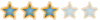Super ZenkaiSuper ZenkaiA forum of the Dragon Ball Universe. Dragon Ball, Dragon Ball Z, and Dragon Ball Super. Even GT.HomeCalendarFAQSearchMemberlistUsergroupsRegisterLog in

 Super Zenkai :: Dragon Ball Discussion :: Dragon Ball Z Discussion Share

# CalculationsAuthorMessage

Super Saiyan RosePosts : 325
Power Level : 976
Reputation : 18
Join date : 2016-02-09
Age : 20Subject: CalculationsTue Jan 31, 2017 7:20 pm 280 to 300= 20 increase177 to 1,480= 1,303 increase250 to 1,830= 1,580 increase206 to 1,770= 1,564 increase140 to 610= 470 increase610 to 31,410= 30,800 increase1,480 to 10,280= 8,7001,480 to 10,583= 9,103 increase1,830 to 10,630= 30,800416 to 8,016= 7,600 increaseCalculations